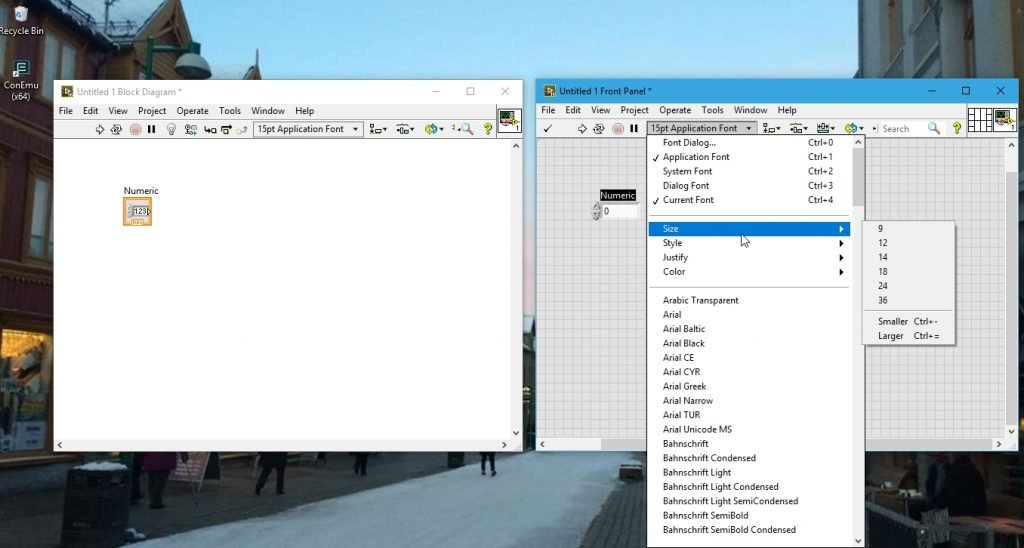# labview programming with examples : tutorial 2

In this tutorial you will learn to write a basic and very simple program or VI and I will provide you with the basic information regarding the blocks and palettes. The first program uses just string constants and indicators and the second program is to do simple addition and subtraction. At the end of each program I have given some exercises and are left for the reader to do it by themselves to make their concepts clear.

### Introduction to labview programming

We have already discussed some of the basic concepts about LabView in previous tutorial. Let’s now move towards programming. Writing a program in LabView is way more simpler than writing the program doing the same functionality in any other programming language i.e. C, C#, Java etc. You need not to learn any of the coding skills or syntax. All you have to do is to make a block diagram and run it. A LabView program is known as a Virtual Instrument (VI). In the entire upcoming tutorials I will use VI instead of LabView programs. When you create a new VI in LabView it will show two windows, one for block diagram which serves for the functional graphical code and other for front panel which serves as the user interface. You build the block diagram of the required task using controls (input devices) and indicators (output devices) in the block diagram window. The front panel is a user interface and the output of your block diagram is displayed on the front panel which includes all the controllable inputs and all output devices. Let’s move toward the programming now.

### writing Your first labview program

Figure 1: Block diagram and front panel

Figure 2: Control placements

• In the similar way we can also place indicators. Placing indicators on front panel will automatically display that on block diagram too.
• The control palette and function palette contains almost everything one can use to interact with the VI and to display graphs or runtime values of a variable. Both these palettes collectively give an entire variety of controls and indicators.
• Every time you drop an item on the panel there is a corresponding terminal that appears on the block diagram. Clicking on the item in front panel will blink the corresponding item o the block diagram, and updating the value in either of the window will update the value in the other. In short both the windows are linked together.
• One can also resize and re color any of these items to their liking, using the panel shown in the figure below.Figure 3: Resizing and Re coloring

Figure 4: Function palette

Now lets see sample examples of labview programs

### 1.Hello! World program in labview

Figure 5: Placement of string indicator

Figure 6: Placement of string constant

Figure 7: Wire connections

Figure 8: String input

Figure 9: Output display

This is the basic program used to understand the working of string constants and indicator and is used as a beginners code all over the world. Now let’s do a simple exercise to make this concept clear.

Exercise:

• Try to display your name and address on 2 separate string indicators

(Hint: Use different string constants and indicators)

### Addition and subtraction program in labview

Trying to do difficult tasks including complex mathematics will somehow always find its way to simple addition and subtractions. If you are not familiar with doing simpler tasks you can never be able to do complex task. Starting up your pace using addition will make you feel that you are learning something. If I start from complex problem where the beginner is not even able to write a simple code after the end of the tutorial then writing these tutorials is of no use. Using numerical operators such as addition, subtraction, multiplication, and division is the key to be good programmer in the future.

Let’s now move towards the programming part and try to do some calculation. At the end I will assign you an exercise to do it by yourself, because learning without practicing is of no use and programming cannot be learned just by reading. You have to write programs and solve a particular task if you are willing to be a good programmer in future.

Figure 10: Placement of Add operator

Figure 11: Placement of numeric constants

Figure 12: Placement of numeric indicator.

Figure 13: Output of addition displayed

Figure 14: Placement of subtraction

Figure 15: Subtraction output

Figure 16: Subtraction help

Now do a small exercise to make your concepts of simple numeric operators and constants clear.

### Exercise to learn Labview programming

• Try to do multiple input addition i.e. give 3 inputs to the add operator and display the output and verify your answer. Similarly do addition for four and five input values.

(Hint: Try using multiple add operators)

• Try to do multiple input subtractions i.e. three four and five input subtraction.

(Hint: Always remember the order of subtraction to avoid ambiguity)

• Try using multiplication as well as division operators.

<<previous tutorial                                                                                          Next tutorial>>

### 2 thoughts on “labview programming with examples : tutorial 2”

1. No one is so rich that they can not help others, and no one is too poor to help others in some way